# Excel calculations: automatic, manual, iterative

The tutorial explains the basics of Excel calculation settings and how to configure them to have formulas recalculated automatically and manually.

To be able to use Excel formulas efficiently, you need to understand how Microsoft Excel does calculations. There are many details you should know about basic Excel formulas, functions, the order of arithmetic operations, and so on. Less known, but no less important are "background" settings that can speed up, slow down, or even stop your Excel calculations.

Overall, there are three basic Excel calculations settings you should be familiar with:

Calculation mode - whether Excel formulas are recalculated manually or automatically.

Iteration - the number of times a formula is recalculated until a specific numeric condition is met.

Precision - the degree of accuracy for a calculation.

In this tutorial, we will have a close look at how each of the above settings works and how to change them.

## Excel automatic calculation vs. manual calculation (calculation mode)

These options control when and how Excel recalculates formulas. When you first open or edit a workbook, Excel automatically recalculates those formulas whose dependent values (cells, values, or names referenced in a formula) have changed. However, you are free to alter this behavior and even stop calculation in Excel.

### How to change Excel calculation options

On the Excel ribbon, go to the Formulas tab > Calculation group, click the Calculation Options button and select one of the following options: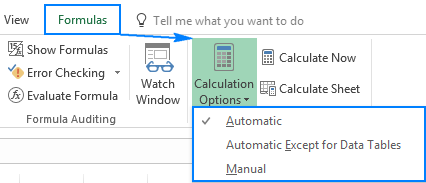Automatic (default) - tells Excel to automatically recalculate all dependent formulas every time any value, formula, or name referenced in those formulas is changed.

Automatic Except for Data Tables - automatically recalculate all dependent formulas except data tables.

Please do not confuse Excel Tables (Insert > Table) and Data Tables that evaluate different values for formulas (Data > What-If Analysis > Data Table). This option stops automatic recalculation of data tables only, regular Excel tables will still be calculated automatically.

Manual - turns off automatic calculation in Excel. Open workbooks will be recalculated only when you explicitly do so by using one of these methods.

Alternatively, you can change the Excel calculations settings via Excel Options:

• In Excel 2010 - Excel 365, go to File > Options > Formulas > Calculation options section > Workbook Calculation.
• In Excel 2007, click Office button > Excel options > Formulas > Workbook Calculation.
• In Excel 2003, click Tools > Options > Calculation > Calculation.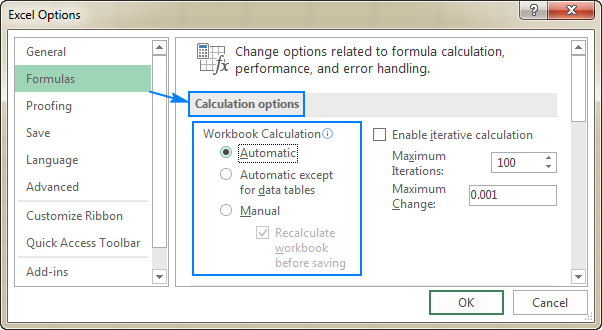Tips and notes:

1. Selecting the Manual calculation option (either on the ribbon or in Excel Options) automatically checks the Recalculate workbook before saving box. If your workbook contains a lot of formulas, you may want to clear this check box to make the workbook save faster.
2. If all of a sudden your Excel formulas have stopped calculating, go to Calculation Options and make sure the Automatic setting is selected. If this does not help, check out these troubleshooting steps: Excel formulas not working, not updating, not calculating.

### How to force recalculation in Excel

If you have turned off Excel automatic calculation, i.e. selected the Manual calculation setting, you can force Excel to recalculate by using one of the following methods.

To manually recalculate all open worksheets and update all open chart sheets, go to the Formulas tab > Calculation group, and click the Calculate Now button.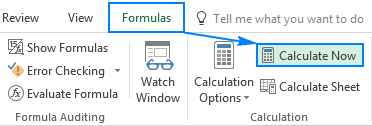To recalculate only the active worksheet as well as any charts and chart sheets linked to it, go to the Formulas tab > Calculation group, and click the Calculate Sheet button.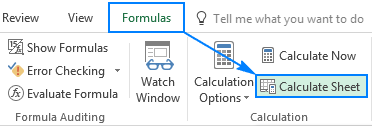Another way to recalculate worksheets manually is by using keyboard shortcuts:

• F9 recalculates formulas in all open workbooks, but only those formulas that have changed since the last calculation and formulas dependent on them.
• Shift + F9 recalculates changed formulas in the active worksheet only.
• Ctrl + Alt + F9 forces Excel to recalculate absolutely all formulas in all open workbooks, even those that have not been changed. When you have the feeling that some formulas are showing incorrect results, use this shortcut to make sure everything has been recalculated.
• Ctrl + Shift + Alt + F9 checks formulas dependent on other cells first, and then recalculates all formulas in all open workbooks, regardless of whether they have changed since the last calculation or not.

## Excel iterative calculation

Microsoft Excel uses iteration (repeated calculation) to compute formulas that refer back to their own cells, which is called circular references. Excel does not calculate such formulas by default because a circular reference can iterate indefinitely creating an endless loop. To enable circular references in your worksheets, you must specify how many times you want a formula to recalculate.

### How to enable and control iterative calculation in Excel

To turn on Excel iterative calculation, do one of the following:

• In Excel 2016, Excel 2013, and Excel 2010, go to File > Options > Formulas, and select the Enable iterative calculation check box under the Calculation options
• In Excel 2007, click Office button> Excel options > Formulas > Iteration area.
• In Excel 2003 and earlier, go to Menu> Tools > Options > Calculation tab > Iterative Calculation.

To change the number of times your Excel formulas can recalculate, configure the following settings:

• In the Maximum Iterations box, type the maximum number of iterations allowed. The higher the number, the more slowly a worksheet is recalculated.
• In the Maximum Change box, type the maximum amount of change between the recalculated results. The smaller the number, the more accurate the result and the longer a worksheet recalculates.

The default settings are 100 for Maximum Iterations, and 0.001 for Maximum Change. It means that Excel will stop recalculating your formulas either after 100 iterations or after a less than 0.001 change between iterations, whichever comes first.

With all the settings configured, click OK to save the changes and close the Excel Options dialog box.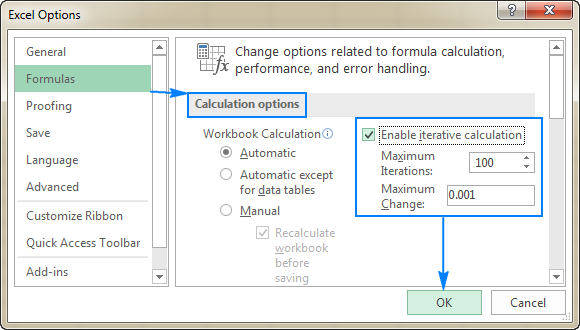## Precision of Excel calculations

By default, Microsoft Excel calculates formulas and stores the results with 15 significant digits of precision. However, you can change this and make Excel use the displayed value instead of the stored value when it recalculates formulas. Before making the change, please be sure you fully understand all possible consequences.

In many cases, a value displayed in a cell and the underlying value (stored value) are different. For example, you can display the same date in a number of ways: 1/1/2017, 1-Jan-2017 and even Jan-17 depending on what date format you set up for the cell. No matter how the display value changes, the stored value remains the same (in this example, it's the serial number 42736 that represents January 1, 2017 in the internal Excel system). And Excel will use that stored value in all formulas and calculations.

Sometimes, the difference between the displayed and stored values can make you think that a formula's result is wrong. For example, if you enter the number 5.002 in one cell, 5.003 in another cell and choose to display only 2 decimal places in those cells, Microsoft Excel will display 5.00 in both. Then, you add up those numbers, and Excel returns 10.01 because it calculates the stored values (5.002 and 5.003), not the displayed values.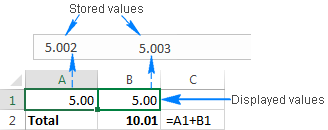Selecting the Precision as displayed option will cause Excel to permanently change stored values to the displayed values, and the above calculation would return 10.00 (5.00 + 5.00). If later on you want to calculate with full precision, it won't be possible to restore the original values (5.002 and 5.003).

If you have a long chain of dependent formulas (some formulas do intermediate calculations used in other formulas), the final result may become increasingly inaccurate. To avoid this "cumulative effect", it stands to reason changing the displayed values via custom Excel number format instead of Precision as displayed.

For example, you can increase or decrease the number of displayed decimal places by clicking the corresponding button on the Home tab, in the Number group: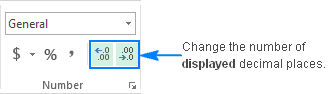### How to set calculation precision as displayed

If you are confident that the displayed precision will ensure the desired accuracy of your Excel calculations, you can turn it on in this way:

1. Click the File tab > Options, and select the Advanced category.
2. Scroll down to the When calculating this workbook section, and select the workbook for which you want to change the precision of calculations.
3. Check the Set precision as displayed box.
4. Click OK.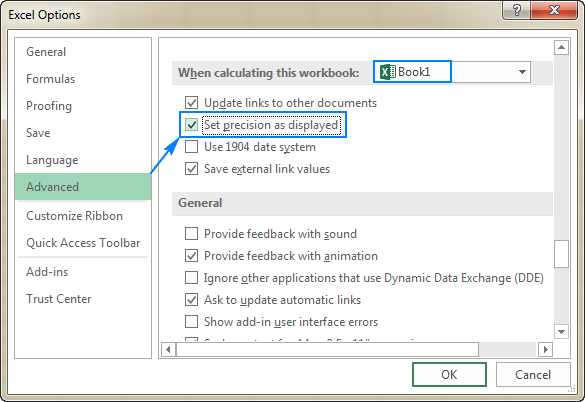This is how you configure calculation settings in Excel. I thank you for reading and hope to see you on our blog next week!

## You may also be interested in

1. I'm trying to solve a slightly different problem for calculations to save time. I keep calculations set to "Manual" and use a button/macro combination to recalculate when necessary. However, I'm seeing behavior you referenced above....namely, with more that one workbook open, the calculations are taking longer. Specifically, I can see that each workbook is calculating.

Here is the code I currently use to refresh the workbook:

Sub RefreshData()
'
' This macro executes when the "Refresh" button is pressed
' RefreshData Macro
' Performs the same action as selecting the "Refresh All" option from the Data menu
'
' Keyboard Shortcut: Ctrl+r
'

Call SetWorkbookCalc(True)
ActiveWorkbook.RefreshAll
Call SetWorkbookCalc(False)

End Sub

Sub SetWorkbookCalc(ByVal bTurnOn As Boolean)

If bTurnOn Then
Application.Calculation = xlAutomatic
Range("WorkbookCalcStatus").Value = "ON"
Else
Range("WorkbookCalcStatus").Value = "OFF"
Application.Calculation = xlManual
End If

End Sub
-------------------------------------------

I typically have three or four excel workbooks open at the same time. There are no references between any of the workbooks and they are completely independent of each other. But when I'm working in one workbook and ready to refresh the calculations, I only want that active workbook (all worksheets in that active workbook!) to refresh. Can you suggest settings or VBA to accomplish such?

• Hi!

2. Any ideas how to consolidate 10 loans. This loans obtain at different time periods in a 3 yr schedule. with different interest rate same amortization time. ? Thoughts?

3. Hello In My Excel I Enter 1000 then come 100 and e just edit then Again 10 afte 0.10 0.010 have somthing changed in execl setting If someone know just let me know plz thank you

4. I am impressed. However, I have a slightly different issue with order of excel calculations. I use VBA to do my calculations in blocks because there are so many components to a single calculation-- for example block 1 result goes into block 2, block 3, block 4, etc. and each of these blocks can take some time because of a high precision addon with matrix inversion I am using. The snag is that block 2 also goes into block 3 so I do not want block 3, 4, etc to recalculate until block 2 has been recalculated, etc. I know what I want can be achieved by switching to manual calculation mode and then using vba to calculate block 1 then block 2 then block 3 etc, but I am wondering whether I need to do that? I am now thinking that maybe Excel will sort that out for me without too much unnecessary calculation.

5. How do I create a formula to have a value entered in one cell to be added in one cell and again be subtracted in another i.e 100 entered in cell B be subtracted from cell A but at the same time be added into cell C

• Hi!
Cells A1 and C1 should contain formulas that refer to B1. If the values are written there, then your problem can only be solved with a VBA macro.

6. Thx. Never knew about automatic/manual setting. Fixed my issue.

7. How can I implement the formula A=A+B in excel?

• Hello Jithin!
Either a formula or a value can be written in an Excel cell. If you entered value in cell A, then you can change this cell either manually or using the VBA.

8. I did observe in salary Bill both manual entry and formula are working , after editing a cell that posted due to formula if post manual entry , manual entry taking in the cell if we removed Manual posting, automatically formula working, how is it possible please explain

9. Is it possible to have 1 formula calculate manually and the rest automatic? i am using =rand in my table but don't want it to calculate everytime.. just when i need it to.

• Hello Paul!
Unfortunately this is not possible. All formulas on the sheet are recounted.
However, I think you can try the following. You transfer the RAND function to a separate file. In your file, place a link to the cell with this function. If this separate file is closed, the RAND function will not be recounted. If you need to recount, just open this separate file in Excel.
If Excel uses an external reference to another file, it tries to recalculate it to get accurate final data. If Excel can’t do this for some reason, it doesn’t consider the file version as final and doesn’t get anything from it.

Thus, if any additional actions are required from a user to recalculate the data in the source file, you won’t get the data from this file until the user opens it and allows to update the data or run macros. Unless the file is open, the references can’t be updated. Therefore, it is not possible to automatically recalculate the data.

10. Ciao Svetlana,
ho bisogno di creare delle celle in cui singolarmente posso inserire solo un carattere
e poi appena inserito il cursore si posiziona automaticamente in quella successiva senza che io premi INVIO.
Come si fa ?

Grazie

• Hello!
You can permit entering just a single character in a cell with the help of Data Validation. To learn more about restricting the length of the text you enter, please see our blog: https://www.ablebits.com/office-addins-blog/data-validation-excel/#text-length

To automatically move the cursor to the next cell after the data is entered, please adjust the Excel options.

11. hi,
if i enter some formula in C2 cell for Ex(=COUNTIFS(C2:C21,"5",D2:D21,"2",E2:E21,"0") ,and i will enter D2 cell in the value of "520",in this case i have to change "520" into "420".

how can, the above C2 cell formula change "5","2","0" into "4","2","0" is there any possibility to change the formula

Thanks

• I want formula for automatic continuous subtraction for collection department.
For example. I disbursed 1000 and i will receive it in 20 steps so in every step received amount entered should automatically deducted from outstanding and so for the next day also

13. DO you know how could I turn off "Recalculate workbook before saving" option with a registry value?

14. Hello,
Please help me to solve my problem. I am preparing the Invoice to my client, I use round and trunc functions but still i am getting error in 2nd decimal...Is there any way where I can do the simple addition and subtraction like calculator in excel

15. I have lots of formulas in my excel like now() and today() which are supposed to give current time and date..And are supposed to change regularly as time elapses. But I can observe in my sheet that the formulas are not changing consistently with time and at times I have to click on "calculate now" to get the current value. I have these cells referenced to other sheets as well..It is very disappointing, is there any solution to this problem a
so that my time dependent formal display consistent result with time ?

16. Hi, Svetlana, i love your tutorial so much,
i have learn many,
Thanks for your sharing, so nice, so useful, so amazing

17. Hi, Svetlana. I'm not sure if this is somehow related to this topic. But I am always getting a different result from a manual computation (like with the formula) of average and the average from a pivot/table. Let say if the result of the manual computation is 98.34, the pivot will have 98.37. Thanks in advance

18. Hello, Svetlana

How to reduce sets of numbers by formula.

My mathematic and excel formula is not strong, I have a large number of sets of numbers DATA A1,A2,C1,C2 each set contains 100 numbers and B1,B2 contain 1 number. each number are given (A1+B1=C1),(A2+B2=C2), C1-9 matching C2-94 and C1-99 matching C2-76. my final result number 9 & 99 = B2 . the formula can be use on DATA A1,A2,C1.